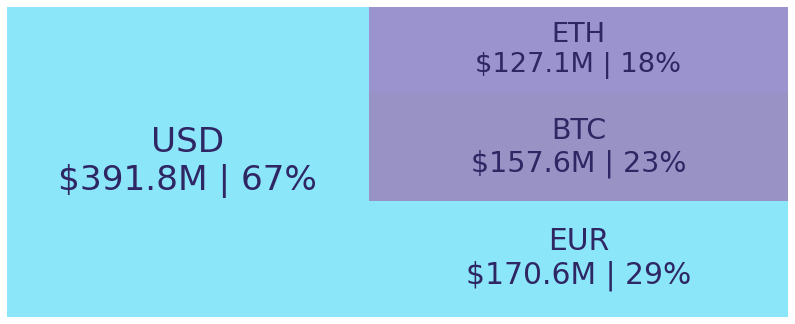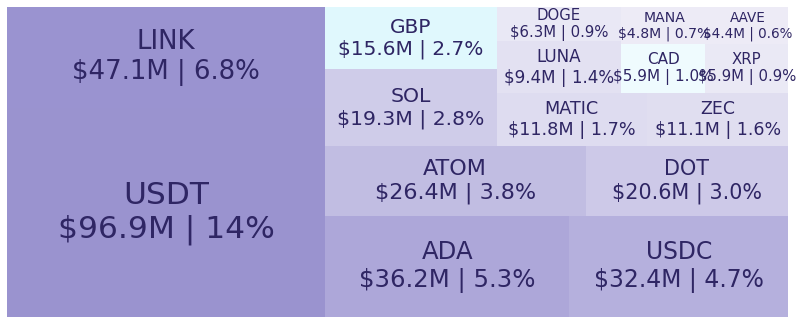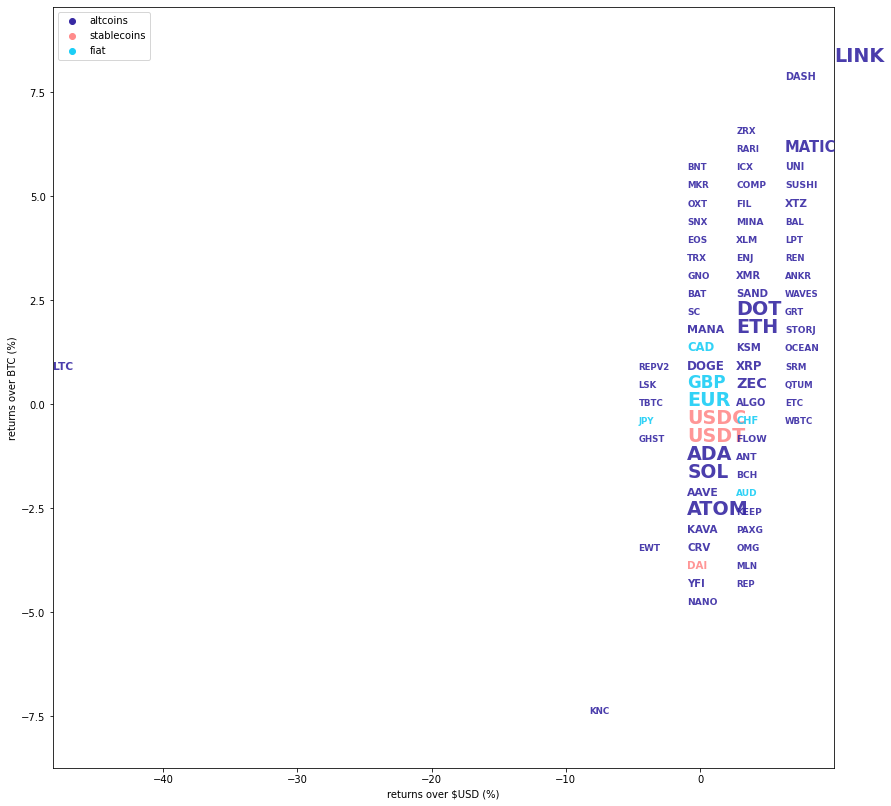# Kraken Daily Market Report for January 09 2022

## Overview

• Total spot trading volume at $638.1 million, the 30 day average is$1.11 billion.
• Total futures notional at $162.0 million. • The top traded coins were, respectively, Bitcoin (+0.4%), Ethereum (+2.3%), Tether (0%), Chainlink (+8.8%), and Cardano (-1.0%). • Good returns from Luna (+11%) and Dash (+7.9%). January 09, 2022$638.1M traded across all markets today
Crypto, EUR, USD, JPY, CAD, GBP, CHF, AUD
BTC
$41862. ↑0.4%$157.6M
ETH
$3150.2 ↑2.3%$127.1M
USDT
$1.0 ↑0.0%$96.9M
$27.535 ↑8.8%$47.1M
$1.1695 ↓1.0%$36.2M
USDC
$1.0001 ↑0.01%$32.4M
ATOM
$35.011 ↓2.2%$26.4M
DOT
$24.607 ↑2.6%$20.6M
SOL
$140.85 ↓0.9%$19.3M
MATIC
$2.1162 ↑6.6%$11.8M
ZEC
$144.13 ↑0.9%$11.1M
LUNA
$74.477 ↑11%$9.37M
DOGE
$0.1504 ↓0.25%$6.26M
XRP
$0.7525 ↑1.0%$5.88M
MANA
$2.9369 ↑0.08%$4.75M
AAVE
$209.46 ↓1.0%$4.4M
LTC
$131.03 ↓47%$4.1M
XTZ
$4.2164 ↑5.2%$3.73M
KAVA
$4.5881 ↓2.4%$3.43M
CRV
$4.5 ↓2.2%$3.38M
DAI
$1.0000 ↓0.01%$3.24M
SAND
$4.724 ↑3.1%$3.11M
YFI
$32658. ↓1.3%$2.96M
KSM
$246.6 ↑2.8%$2.74M
AVAX
$89.21 ↑6.4%$2.73M
ALGO
$1.401 ↑1.2%$2.47M
XMR
$191.0 ↑3.7%$2.46M
DASH
$153.34 ↑7.9%$2.27M
UNI
$15.788 ↑5.9%$2.09M
LRC
$1.5757 ↑3.9%$2.06M
FLOW
$7.8 ↑1.7%$1.78M
SUSHI
$6.93 ↑6.0%$1.65M
ANT
$8.2075 ↑1.6%$1.53M
ENJ
$2.298 ↑1.9%$1.43M
XLM
$0.2611 ↑2.7%$1.33M
MINA
$3.35 ↑3.7%$1.29M
FIL
$30.24 ↑2.6%$1.1M
NANO
$3.0073 ↓1.4%$1.01M
BCH
$377.02 ↑1.1%$999K
SHIB
$2.828e ↑1.9%$931K
SC
$0.0134 ↑1.7%$912K
COMP
$194.27 ↑3.3%$888K
MOVR
$160.33 ↑2.6%$871K
STORJ
$1.5580 ↑3.2%$860K
ICX
$1.0147 ↑3.4%$853K
BAT
$1.0346 ↑1.4%$783K
KEEP
$0.6242 ↑1.9%$741K
EWT
$7.759 ↓3.5%$722K
GNO
$472.0 ↑1.4%$705K
TRX
$0.0661 ↑1.2%$664K
PAXG
$1797.4 ↓0.16%$654K
EOS
$2.7946 ↑1.7%$647K
OCEAN
$0.7396 ↑2.3%$560K
BAL
$18.71 ↑4.9%$508K
KNC
$1.4241 ↓6.8%$488K
SDN
$1.454 ↑7.4%$474K
SNX
$5.0827 ↑1.5%$386K
KILT
$3.15 ↑1.3%$338K
AXS
$71.883 ↑2.5%$332K
LPT
$37.01 ↑6.5%$317K
OXT
$0.3159 ↑1.2%$309K
PERP
$9.203 ↑2.1%$307K
OMG
$5.3537 ↓0.11%$299K
GRT
$0.5842 ↑2.3%$276K
RARI
$13.67 ↑1.6%$261K
MLN
$75.647 ↑1.5%$260K
WAVES
$14.096 ↑3.1%$249K
QTUM
$7.6649 ↑1.5%$249K
CTSI
$0.5853 ↑1.8%$231K
LSK
$2.0530 ↑0.1%$231K
CHZ
$0.2617 ↑2.5%$199K
SRM
$3.028 ↑1.8%$187K
INJ
$6.737 ↓0.7%$183K
DYDX
$6.813 ↑1.9%$180K
ETC
$29.931 ↑2.0%$151K
WBTC
$42568. ↑2.6%$142K
MKR
$2126.2 ↑0.8%$140K
ANKR
$0.0928 ↑2.4%$97.3K
CQT
$0.515 ↓1.7%$96.8K
$12.201 ↓0.16%$88.1K
KAR
$3.15 ↑5.0%$82.2K
PHA
$0.359 ↑2.0%$79.5K
ZRX
$0.726 ↑2.1%$77.5K
REN
$0.4654 ↑5.4%$74.4K
REP
$16.495 ↑1.4%$69.1K
BAND
$5.336 ↑8.9%$60.1K
BNC
$1.4366 ↑0.5%$52.3K
OXY
$0.549 ↑3.0%$49.4K
1INCH
$2.144 ↑1.2%$47.9K
GHST
$2.1257 ↑0.21%$31.5K
RAY
$5.364 ↑0.9%$30.6K
MIR
$1.846 ↓0.16%$21.9K
OGN
$0.5262 ↑1.6%$18.5K
BNT
$3.169 ↑1.4%$16.0K
REPV2
$16.245 ↑0.19%$12.9K
TBTC
$42465. ↓1.0%$4.92K

#####################. Trading Volume by Asset. ##########################################

The figures below break down the trading volume of the largest, mid-size, and smallest assets. Cryptos are in purple, fiats are in blue. For each asset, the chart contains the daily trading volume in USD, and the percentage of the total trading volume. The percentages for fiats and cryptos are treated separately, so that they both add up to 100%.

Figure 1: Largest trading assets: trading volume (measured in USD) and its percentage of the total trading volume (January 09 2022)Figure 2: Mid-size trading assets: (measured in USD) (January 09 2022)###########. Daily Returns. #################################################

## Daily Returns %

Figure 3: Returns over USD and XBT. Relative volume and return size is indicated by the size of the font. (January 09 2022)###########. Disclaimer #################################################

The values generated in this report are from public market data distributed from Kraken WebSockets api. The total volumes and returns are calculated over the reporting day using UTC time.